# Samacheer Kalvi Books: Tamilnadu State Board Text Books Solutions

## Samacheer Kalvi 10th Maths Chapter 5 Coordinate Geometry Notes PDF Download: Tamil Nadu STD 10th Maths Chapter 5 Coordinate Geometry NotesSamacheer Kalvi 10th Maths Chapter 5 Coordinate Geometry Notes PDF Download: Tamil Nadu STD 10th Maths Chapter 5 Coordinate Geometry Notes

## Samacheer Kalvi 10th Maths Chapter 5 Coordinate Geometry Notes PDF Download

We bring to you specially curated Samacheer Kalvi 10th Maths Chapter 5 Coordinate Geometry Notes PDF which have been prepared by our subject experts after carefully following the trend of the exam in the last few years. The notes will not only serve for revision purposes, but also will have several cuts and easy methods to go about a difficult problem.

 Board Tamilnadu Board Study Material Notes Class Samacheer Kalvi 10th Maths Subject 10th Maths Chapter Chapter 5 Coordinate Geometry Format PDF Provider Samacheer Kalvi Books

## How to Download Samacheer Kalvi 10th Maths Chapter 5 Coordinate Geometry Notes PDFs?

2. Click on the Samacheer Kalvi 10th Maths Notes PDF.
3. Look for your preferred subject.
4. Now download the Samacheer Kalvi 10th Maths Chapter 5 Coordinate Geometry notes PDF.

## Tamilnadu Samacheer Kalvi 10th Maths Solutions Chapter 5 Coordinate Geometry Unit Exercise 5

Question 1.
PQRS is a rectangle formed by joining the points P(-1, -1), Q(-1, 4) ,R(5, 4) and S(5, -1). A, B, C and D are the mid-points of PQ, QR, RS and SP respectively. Is the quadrilateral ABCD a square, a rectangle or a rhombus? Justify your answer.
Solution:
A, B, C and D are mid points of PQ, QR, RS & SP respectively.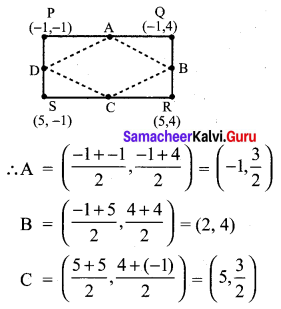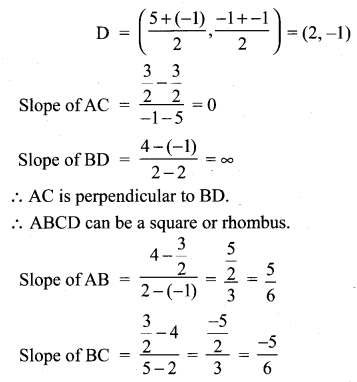∴ AB and BC are not perpendicular
⇒ ABCD is rhombus as diagonals are perpendicular and sides are not perpendicular.

Question 2.
The area of a triangle is 5 sq.units. Two of its vertices are (2, 1) and (3, -2). The third Vertex is (x, y) where y = x + 3 . Find the coordinates of the third vertex.
Solution:
Area of triangle formed by points (x1, y1),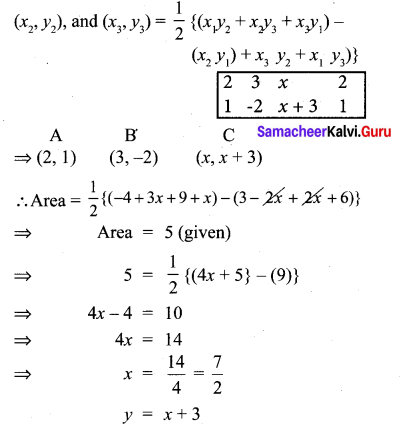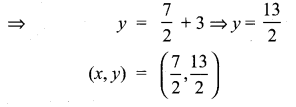Question 3.
Find the area of a triangle formed by the lines 3x + y – 2 = 0, 5x + 2y – 3 = 0 and 2x – y – 3 = 0
Solution: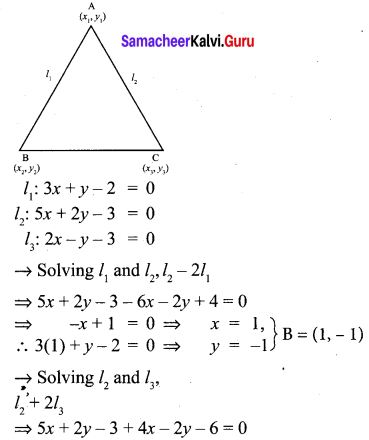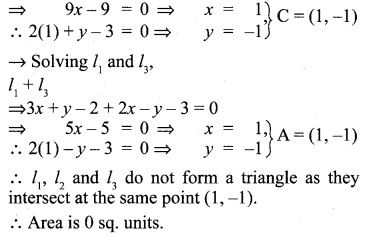Question 4.
If vertices of a quadrilateral are at A(-5, 7), B(-4, k) , C(-1, -6) and D(4, 5) and its area is
72 sq.units. Find the value of k.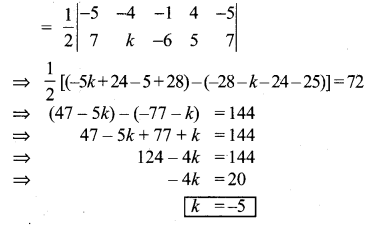Question 5.
Without using distance formula, show that the points (-2, -1) , (4, 0) , (3, 3) and (-3, 2) are vertices of a parallelogram.
Solution: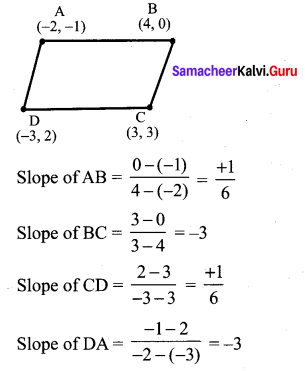Slope of AB = Slope of CD
Slope of BC = Slope of DA
Hence ABCD forms a parallelogram.

Question 6.
Find the equations of the lines, whose sum and product of intercepts are 1 and -6 respectively.
Let the intercepts be x1, y1 respectively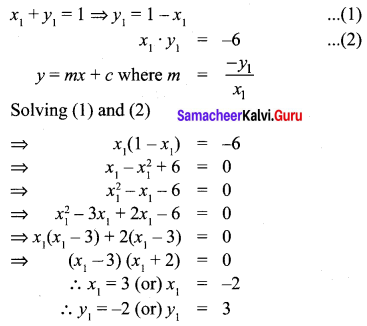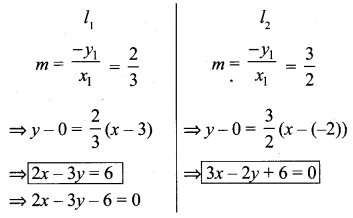Question 7.
The owner of a milk store finds that, he can sell 980 litres of milk each week at ₹ 14/litre and 1220 litres of milk each week at ₹ 16 litre. Assuming a linear relationship
between selling price and demand, how many litres could he sell weekly at ₹ 17/litre?
Solution: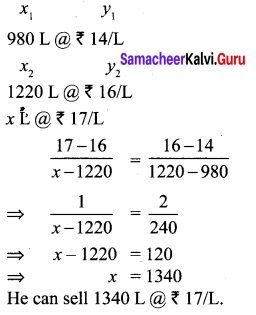Question 8.
Find the image of the point (3, 8) with respect to the line x + 3y = 7 assuming the line to be a plane mirror.
Solution: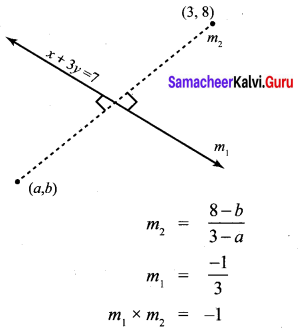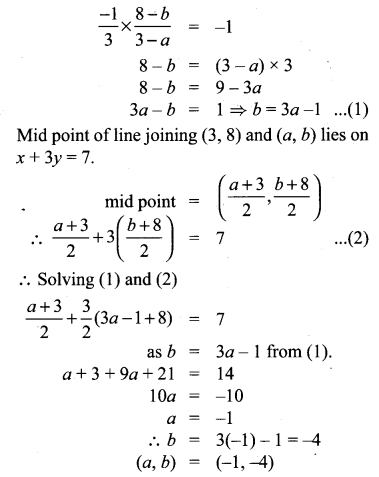Question 9.
Find the equation of a line passing through the point of intersection of the lines 4x + 7y – 3 = 0 and 2x – 3y + 1 = 0 that has equal intercepts on the axes.
Solution:
4x + 7y – 3 = 0
2x – 3y + 1 = 0
4x + 7y – 3 – 2(2x – 3y + 1) = 0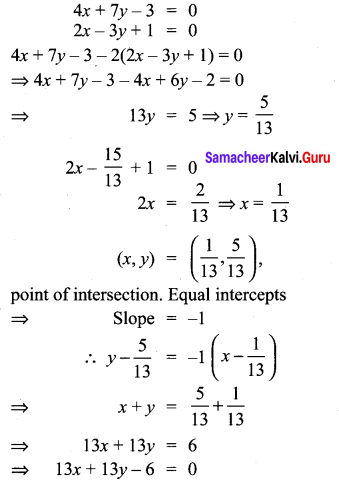Question 10.
A person standing at a junction (crossing) of two straight paths represented by the equations 2x – 3y + 4 = 0 and 3x + 4y – 5 = 0 seek to reach the path whose equation is 6x – 7y + 8 = 0 in the least time. Find the equation of the path that he should follow.
Solution: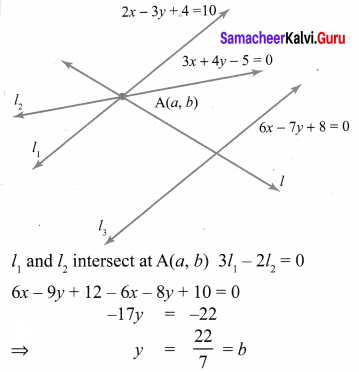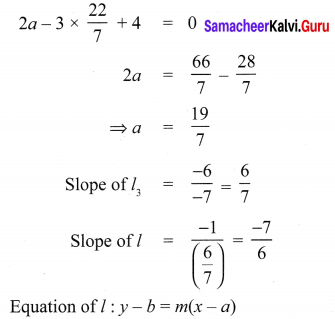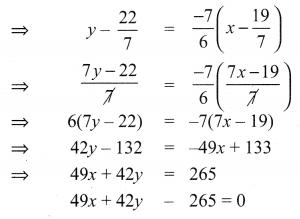## Tamilnadu Samacheer Kalvi 10th Maths Solutions Chapter 5 Coordinate Geometry Additional Questions

Question 1.
Find a relation between x and y such that the point (x, y) is equidistant from the points (7, 1) and (3, 5).
Solution:
Let P(x, y) be equidistant from the points A(7, 1) and B(3, 5).
We are given that AP = BP. So, AP2 = BP2
(x – 7)2 + (y – 1)2 =(x – 3)2 + (y – 5)2
x2 – 14x + 49 + y2 – 2y + 1 = x2 – 6x + 9 + y2 – 10y + 25
x – y = 2
Which is the required relation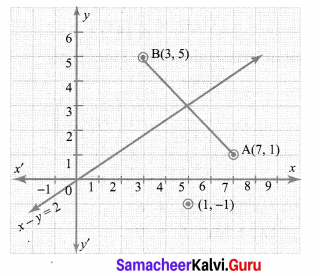Question 2.
Show that the points (1, 7), (4, 2), (-1, -1) and (-4, 4) are the vertices of a square.
Solution:
Let A(1, 7), B(4, 2), C(-1, -1) and D(-4, 4) be the given points. To prove that ABCD is a square, we have to prove that all its sides are equal and both its diagonals are equal.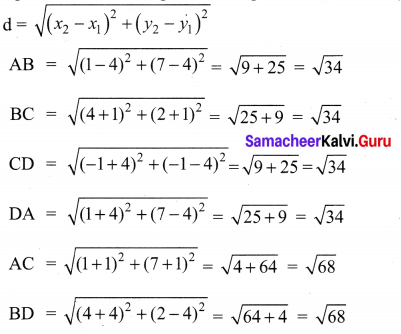Since, AB = BC = CD = DA and AC = BD, all the four sides of the quadrilateral ABCD are equal and its diagonals AC and BD are also equal. Therefore, ABCD is a square.

Question 3.
If A (-5, 7), B (-4, -5), C (-1, -6) and D (4, 5) are the vertices of a quadrilateral, find the area of the quadrilateral ABCD.
Solution:
By joining B to D, you will get two triangles ABD and BCD.
Now, the area of ∆ABD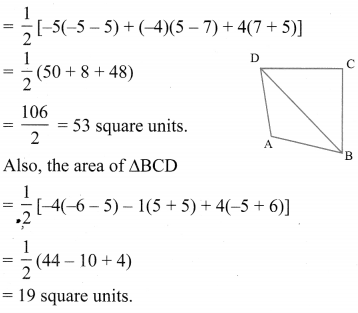So, the area of quadrilateral ABCD = 53 + 19 = 72 square units.

Question 4.
Find the coordinates of the points of trisection (i.e. points dividing in three equal parts) of the line segment joining the points A(2, -2) and B(-7, 4).
Solution:
Let P and Q be the points of trisection at AB. i.e., AP = PQ = QB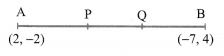Therefore, P divides AB internally in the ratio 1 : 2. Therefore, the coordinates at P, by applying the section formula, are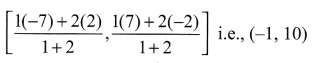Now, Q also divides AB internally in the ratio 2 : 1, so, the coordinates at Q are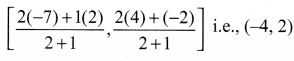Therefore, the coordinates of the points at trisection of the line segment joining A and B are (-1, 0) and (-4, 2).

Question 5.
If the points A(6, 1), B(8, 2), C(9, 4) and D(P, 3) are the vertices of a parallelogram, taken in order. Find the value of P.
Solution:
We know that diagonals of a parallelogram bisect each other.
So, the coordinates at the mid-point of AC = coordinates of the mid-point of BD.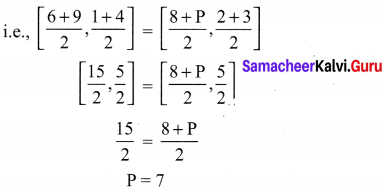Question 6.
Find the area of a triangle whose vertices are (1,-1), (-4, 6) and (-3, -5).
Solution:
The area of the triangle formed by the vertices A(1, -1), B(-4, 6) and C(-3, -5), by using the formula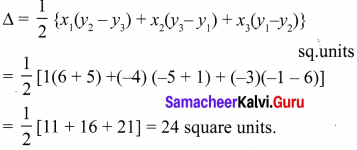So, the area of the triangle is 24 square units.

Question 7.
If A(-2, -1), B(a, 0), C(4, b) and D(1, 2) are the vertices of a parallelogram, find the values of a and b.
Solution:
We know that the diagonals of a parallelogram bisect each other. Therefore the co-ordinates of the midpoint of AC are same as the co-ordinates of the mid-point of BD. i.e.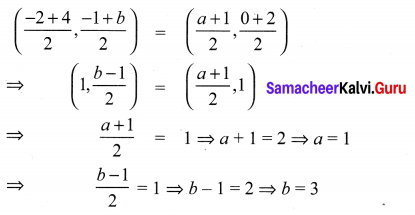Question 8.
Find the area of the quadrilateral whose vertices, taken in order, are (-3, 2), (5, 4), (7, -6) and (-5, -4).
Solution:
We have Area of the quadrilateral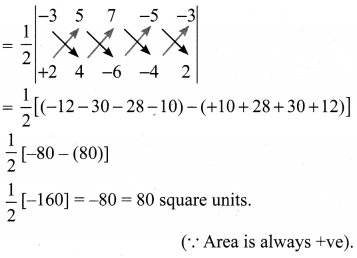Question 9.
Find the area of the triangle formed by the points P(-1.5, 3), Q(6, -2) and R(-3, 4).
Solution:
The area of the triangle formed by the given points is equal to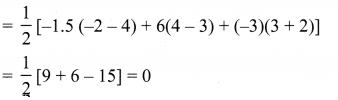Can we have a triangle of area 0 square units? What does this mean?
If the area of a triangle is 0 square units, then its vertices will be collinear.

Question 10.
Find the value of k if the pointsA(2, 3), B(4, k) and (6, -3) are collinear.
Since the given points are collinear, the area a the triangle formed by them must be 0, i.e.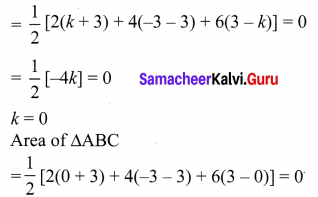## How to Prepare using Samacheer Kalvi 10th Maths Chapter 5 Coordinate Geometry Notes PDF?

Students must prepare for the upcoming exams from Samacheer Kalvi 10th Maths Chapter 5 Coordinate Geometry Notes PDF by following certain essential steps which are provided below.

• Use Samacheer Kalvi 10th Maths Chapter 5 Coordinate Geometry notes by paying attention to facts and ideas.
• Pay attention to the important topics
• Refer TN Board books as well as the books recommended.
• Correctly follow the notes to reduce the number of questions being answered in the exam incorrectly
• Highlight and explain the concepts in details.

## Frequently Asked Questions on Samacheer Kalvi 10th Maths Chapter 5 Coordinate Geometry Notes

#### How to use Samacheer Kalvi 10th Maths Chapter 5 Coordinate Geometry Notes for preparation??

Read TN Board thoroughly, make separate notes for points you forget, formulae, reactions, diagrams. Highlight important points in the book itself and make use of the space provided in the margin to jot down other important points on the same topic from different sources.

#### How to make notes for Samacheer Kalvi 10th Maths Chapter 5 Coordinate Geometry exam?

Read from hand-made notes prepared after understanding concepts, refrain from replicating from the textbook. Use highlighters for important points. Revise from these notes regularly and formulate your own tricks, shortcuts and mnemonics, mappings etc.
Share: